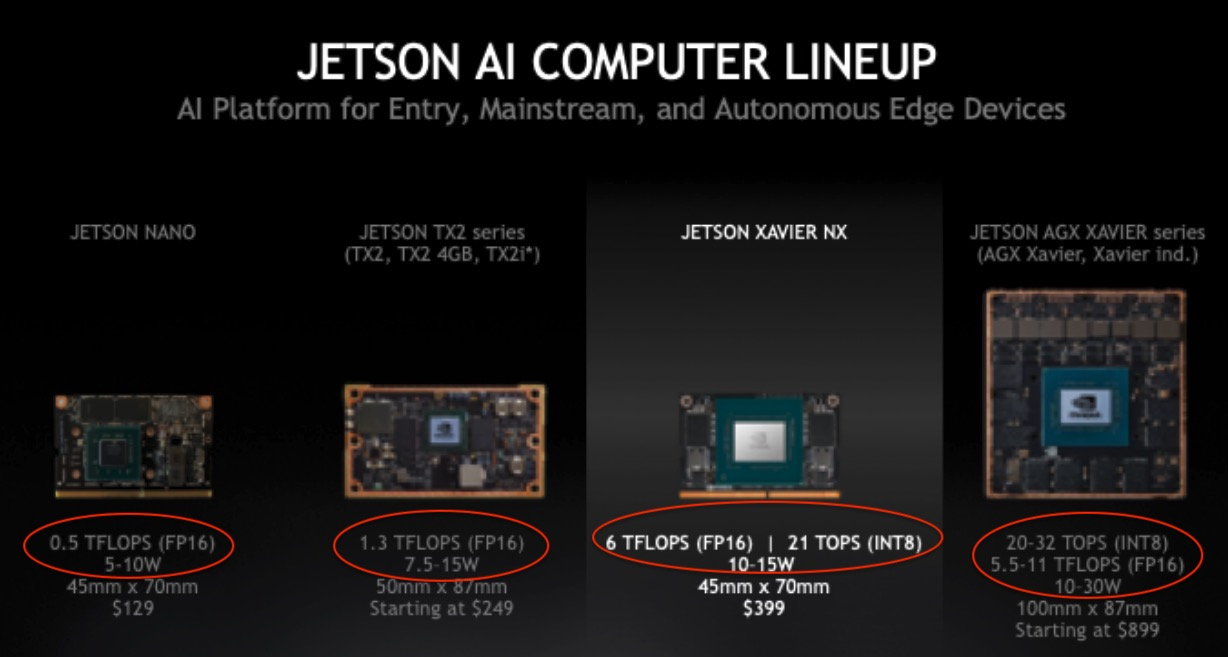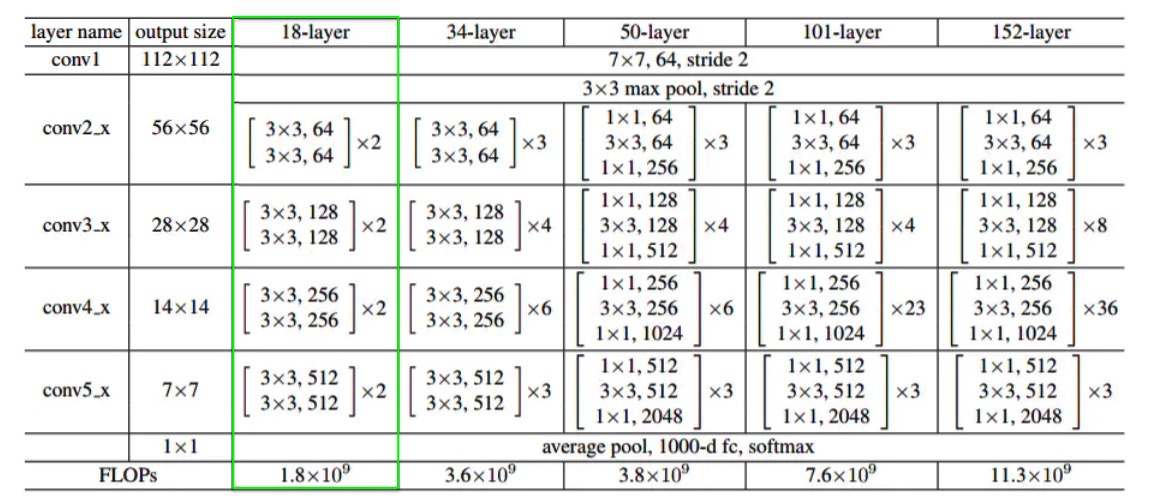# 卷积神经网络的参数量和计算量

《卷积神经网络的参数量和计算量》

对于在端部署的模型来说，模型的参数量以及计算量是锱铢必较的，使用什么样的激活函数，会有多大的计算成本。对于模型的压缩量化等都是模型的参数量和计算量的准确计量为基础的，所以本文简单记录一下常见神经网络的参数量和计算量。

Key Words：Parameters count、FLOPS、FLOPs

Beijing, 2020

Agile Pioneer

• FLOPS (Floating point of per Second) 的缩写，意指每秒浮点运算次数。用来衡量硬件的性能。

• FLOPs (Floating point of operations) 的缩写，是浮点运算次数，可以用来衡量算法/模型复杂度。

## 模型的参数量

• K h K_h 卷积核的高度
• K w K_w 卷积核的宽度
• C i n C_{in} 输入featureMap的channel数
• C o u t C_{out} 输出featureMap的channel数

### 全连接参数量

全连接层的参数量是很大的，和输入的隐藏层的数据大小相关，如VGG-16最后一次卷积得到的feature map的维度为：7x7x512，将feature map展开后输出为4096个隐藏层单元，那么相当于用4096个1x1的卷积对7x7x512展平的结果去做卷积。

7 × 7 × 512 × 4096 = 102760448 7 \times 7 \times 512 \times 4096 = 102760448

### 池化层

对于池化层而言，常用的Max-pooling，Avg-pooling等是不存在参数量的。

### Vanilla Conv Parameters

对于某一个卷积层，它的parameters个数为：

( K h ∗ K w ∗ C i n ) ∗ C o u t + C o u t (K_h * K_w * C_{in}) * C_{out} + C_{out}

### Group Convolution

分组卷积的输出特征图的每个通道，只和输入特征图的一部分通道有关，而这部分通道，就是一个分组(Group)。依旧假设输入特征图的尺寸为 C i n ∗ H ∗ W C_{in} * H * W ，分为 g g 组进行分组卷积，那么，对于每一组，输出特征图的通道数都是 C o u t g \frac{C_{out}}{g} 每组的卷积核参数变为:

C i n g ∗ K h ∗ K w ∗ C o u t g \frac{C_{in}}{g} * K_h * K_w * \frac{C_{out}}{g}

C i n g ∗ K h ∗ K w ∗ C o u t g ∗ g \frac{C_{in}}{g} * K_h * K_w * \frac{C_{out}}{g} * g

### Depthwise Separable Convolution

对于深度可分离卷积，包含两个部分，Depthwise和Point_wise。

Depthwise:

K h ∗ K w ∗ C i n K_h * K_w * C_{in}

Pointwise:

1 ∗ 1 ∗ C i n ∗ C o u t 1 * 1 * C_{in} * C_{out}

final:

K h ∗ K w ∗ C i n + 1 ∗ 1 ∗ C i n ∗ C o u t K_h * K_w * C_{in} + 1 * 1 * C_{in} * C_{out}

K h ∗ K w ∗ C i n + C i n ∗ C o u t ( K h ∗ K w ∗ C i n ) ∗ C o u t + C o u t = 1 C o u t + 1 K h ∗ K w \frac{K_h * K_w * C_{in} + C_{in} * C_{out}}{(K_h * K_w * C_{in}) * C_{out} + C_{out}} = \frac{1}{C_{out}} +\frac{1}{K_h * K_w}

为了便于理解、便于和分组卷积类比，假设 C i n = C o u t C_{in} = C_{out} 。深度卷积其实就是$g = C_{in} = C_{out}$的分组卷积，只不过没有直接将 g g 组结果拼接，所以Depthwise卷积参数量是标准卷积的 1 C o u t \frac{1}{C_{out}} ，而Pointwise卷积的参数量是标注卷积的 1 K h ∗ K w \frac{1}{K_h * K_w}

## 模型的计算量

• FLOPS 注意全部大写 是floating point of per Second 的缩写，意指每秒浮点运算次数。用来衡量硬件的性能。
• FLOPs 是floating point of operations的缩写，是浮点运算次数，可以用来衡量算法/模型复杂度。
• 1 MAC = 2 FLOPs 2 * (卷积乘法 + 卷积乘法自后所有数相加 - 1)（n个数相加只需要n-1次相加，所以无bias -1，有bias +1抵消）细节
• 运算量乘以的图像feature map的维度是 Output feature map的维度
• 加、减、乘、除、指数运算、平方根、最大值…都是一个FLOP

### 卷积运算量（FLOPs）

#### Vanilla Conv

F L O P s = [ （ K h ∗ K w ∗ C i n ) + ( K h ∗ K w ∗ C i n − 1 ） ] ∗ H o u t ∗ W o u t ∗ C o u t FLOPs =[（K_h * K_w * C_{in}) + (K_h * K_w * C_{in} - 1）]* H_{out} * W_{out} * C_{out}

F L O P s = （ 2 ∗ K h ∗ K w ∗ C i n ） ∗ H o u t ∗ W o u t ∗ C o u t FLOPs =（2 * K_h * K_w * C_{in}）* H_{out} * W_{out} * C_{out}

• 参数量 x 输出的特征映射图的 H，W，其他卷积一样可以这样运算

### 全连接层

F L O P s = ( 2 × I − 1 ) × O FLOPs = (2 \times I - 1) \times O

• which I I = input neuron numbers, O O = output neuron numbers
• 同样的 I I 表示乘法 I − 1 I - 1 表示加法

### 池化层

ps: 自己的理解

#### 最大池化

H o u t ∗ W o u t H_{out} * W_{out}

#### 平均池化

( S h ∗ S w − 1 + 1 ) ∗ H o u t ∗ W o u t (S_h * S_w - 1 + 1) * H_{out} * W_{out}

### 激活层

神经网络中是一定存在非线性的激活函数的，比如ReLU或Sigmoid等，当然这些激活函数也是需要时间来计算的，但是由于激活函数一般没有乘法，所以不计算MAC只计算FLOPs

#### ReLU/PReLU/ELU

F L O P s = H o u t × W o u t × C o u t FLOPs = H_{out} \times W_{out} \times C_{out}

#### Sigmoid

在计算FLOPS的时候，我们通常计算加、减、乘、除、指数、平方根等等，作为一个单个的FLOP。因此在Sigmoid中有4个明显的操作，0-x, exp(x), 1 + x, 1 / x

y = 1 1 + e x p ( − x ) y = \frac{1}{1 + exp(-x)}

FLOPs:

F L O P s = H o u t × W o u t × C o u t × 4 FLOPs = H_{out} \times W_{out} \times C_{out} \times 4

实际上不会计算激活函数的计算量的，因为和神经网络的大量乘加运算相比，它占的比例微不足道。所以 we’ll simply assume that the activation function is free.

## 设备FLOPS参考## 以ResNet18为例计算FLOPs• 公式： H o u t ∗ W o u t ∗ K w ∗ K h ∗ C i n ∗ C o u t H_{out} * W_{out} * K_w * K_h * C_{in} * C_{out} (注意：不要忘了resnet的shortcut的add操作)

• c o n v 1 ： 112 ∗ 112 ∗ 7 ∗ 7 ∗ 3 ∗ 64 conv1：112*112*7*7*3*64

• c o n v 2 x ： 56 ∗ 56 ∗ 64 ∗ 64 ∗ 9 + 56 ∗ 56 ∗ 64 ∗ 64 ∗ 9 + 56 ∗ 56 ∗ 64 ∗ 64 conv2x：56*56*64*64*9+56*56*64*64*9+56*56*64*64

• c o n v 3 x ： 28 ∗ 28 ∗ 64 ∗ 128 ∗ 9 + 28 ∗ 28 ∗ 128 ∗ 128 ∗ 9 + 28 ∗ 28 ∗ 64 ∗ 128 conv3x：28*28*64*128*9+28*28*128*128*9+28*28*64*128

• c o n v 4 x ： 14 ∗ 14 ∗ 128 ∗ 256 ∗ 9 + 14 ∗ 14 ∗ 256 ∗ 256 ∗ 9 + 14 ∗ 14 ∗ 128 ∗ 256 conv4x：14*14*128*256*9+14*14*256*256*9+14*14*128*256

• c o n v 5 x ： 7 ∗ 7 ∗ 256 ∗ 512 ∗ 9 + 7 ∗ 7 ∗ 512 ∗ 512 ∗ 9 + 7 ∗ 7 ∗ 256 ∗ 512 conv5x：7*7*256*512*9+7*7*512*512*9+7*7*256*512

• f c ： 512 ∗ 1000 fc：512*1000

总共加起来乘以2，为1.8G flops

## 参考

07-1411万+
07-0948710-03215
07-083341
06-241652
11-2922万+
08-159930
05-14141
05-026484
06-142055
06-081445
09-089万+
11-162万+
05-082万+
03-26171
11-201万+
01-203万+
05-285万+
05-041801